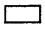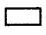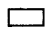Practice the AP 6th Class Maths Bits with Answers Chapter 2 Whole Numbers on a regular basis so that you can attempt exams with utmost confidence.

## AP State Syllabus 6th Class Maths Bits 2nd Lesson Whole Numbers with Answers

I. Choose the correct answer and write it in the brackets.

Question 1.
Whole numbers are represented by
A) N
B) V
C) W
D) O
C) W

Question 2.
Successor of the smallest whole number is ………………..
A) 0
B) 1
C) 10
D) 9
B) 1Question 3.
Are all natural numbers are whole numbers?
A) Yes
B) No
C) We can’t say
D) None
A) Yes

Question 4.
If 5 and 3 are whole numbers, then their sum is ………………
A) Whole number
B) Natural number
C) Negative number
D) None
A) Whole number

Question 5.
Sum of any two whole numbers is always a whole number. This property is called ……………..
A) Commutative
B) Associative
C) Closure
D) None
C) Closure

Question 6.
Subtraction of whole numbers is ………………
A) Closed
B) Not Closed
C) Can’t say
D) None
B) Not Closed

Question 7.
5 × 4 = 4 × 5 this property is called
A) Closure
B) Associative
C) Commutative
D) B and C
C) Commutative

Question 8.
6 – 99 – 6
A) =
B) ≠
C) 0
D) None
B) ≠

Question 9.
7 + 33 + 7
A) =
B) ≠
C) >
D) <
A) =Question 10.
(5 + 4) + 3 = 5 + (4 + 3), this property is called ………………
A) Closure
B) Commutative
C) Associative
C) Associative

Question 11.
(3 + 4) + 5 = 3 + (4 + 5), this property is called …………………
A) Closure
B) Commutative
C) Associative
D) None
C) Associative

Question 12.
If a, b, c are whole numbers the distributive property of multiplication over addition is ……………
A) a + (b × c)
B) a × b + a × c
C) a × (b + c)
D) c = b
D) c = b

Question 13.
A) 1
B) 0
C) 2
D) 4
B) 0

Question 14.
a × (b – c) = (a × b) – (a × c), this property is ………………
A) Distributive of multiplication over addition.
B) Distributive of multiplication over subtraction.
D) Associative over subtraction.
B) Distributive of multiplication over subtraction.

Question 15.
{Whole numbers} – {Natural numbers} = ………………
A) Whole number
B) Natural number
C) A and B
D) None
A) Whole number

Question 16.
We can always form a ……………… with two points.
A) Line
B) Rectangle
C) Triangle
D) None
A) LineQuestion 17.
65 × 99999 = ……………….
A) 64935
B) 644935
C) 649335
D) 6499935
D) 6499935

Question 18.
123456 × 8 + 6 = ………………..
A) 987654
B) 9876543
C) 876543
D) 98765
A) 987654

Question 19.
Number of whole numbers between 0 and 50 is ………………..
A) 49
B) 50
C) 51
D) 52
A) 49

Question 20.
91 × 11 × 5 =
A) 4004
B) 5005
C) 3003
D) 6006
B) 5005

II. Fill in the blanks.

1. Smallest number in W is ………………..
0

2. Predecessor of 1 is …………………..
0

3. Are all whole numbers natural numbers? …………………
No

4. If 5 and 4 are whole numbers, then of (5 – 4) is a ………………. number.
Whole5. Whole numbers W = ………………..
{0, 1, 2, 3, 4, …………..}

6. The value of a number from 0 to its right ……………….
Increases

7. 8 + 9 = 9 + 8 is called ……………….

8. (5 × 6) × 2 = 5 × (6 × 2) is called ………………..
Associative over Multiplication

9. (a × b) + (a × c) = ……………….
a × (b + c)

10. Multiplicative identity is ………………
1

11. Division by zero is ……………….
not defined

12. If
143 × 7 × 1 = 1001
143 × 7 × 2 = 2002
143 × 7 × 9 = ………………
900913. How many 7’s are needed to get 161?
23

14. 99999 × 0 = ………………
0

15. 13680347 × 9 = ……………
123123123

16. a – bb – a

17. Example for a rectangle number ………………
2 × 3 (or) 3 × 2 (or) 2 × 4 (or) 4 × 3, etc

18. Predecessor of smallest whole number is ……………..
0

19. 1111 × 1111 = ……………..
1234321III. Which of the statements are True (T) and which are False (F)?

i) Zero is the smallest whole number.
True

ii) There is a natural number that has no predecessor.
True

iii) All whole numbers are natural numbers.
False

iv) We can show the greatest whole number on the number line.
False

v) A whole number that lies on the number line lies to the right side of another number is the greater number.
True

vi) We can’t show the smallest whole number on the number line.
False

Falseviii) A whole number on the left of another number on the number line is greater.